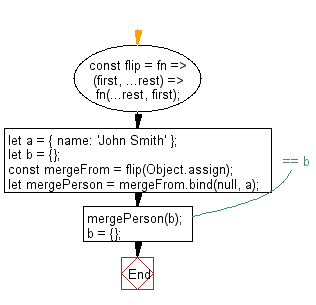# JavaScript: Takes a function as an argument, then makes the first argument the last

## JavaScript fundamental (ES6 Syntax): Exercise-232 with Solution

Write a JavaScript program that takes a function as an argument, then makes the first argument the last.

• Use argument destructuring and a closure with variadic arguments.
• Splice the first argument, using the spread operator (...), to make it the last before applying the rest.

Sample Solution:

JavaScript Code:

``````//#Source https://bit.ly/2neWfJ2
const flip = fn => (first, ...rest) => fn(...rest, first);
let a = { name: 'John Smith' };
let b = {};
const mergeFrom = flip(Object.assign);
let mergePerson = mergeFrom.bind(null, a);
mergePerson(b); // == b
b = {};
console.log(Object.assign(b, a)); // == b
```
```

Sample Output:

```{"name":"John Smith"}
```

Flowchart:Live Demo:

See the Pen javascript-basic-exercise-232-1 by w3resource (@w3resource) on CodePen.

Improve this sample solution and post your code through Disqus

What is the difficulty level of this exercise?

Test your Programming skills with w3resource's quiz.

﻿